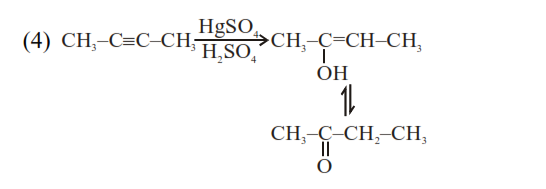# Which one of the following carbonyl compounds cannot be prepared`
Question:

Which one of the following carbonyl compounds cannot be prepared by addition of water on an alkyne in the presence of $\mathrm{HgSO}_{4}$ and $\mathrm{H}_{2} \mathrm{SO}_{4}$ ?

1.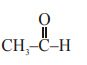2.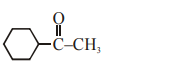3.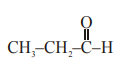4.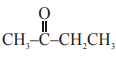Correct Option: , 3

Solution:

Reaction of $\mathrm{HgSO}_{4} /$ dil. $\mathrm{H}_{2} \mathrm{SO}_{4}$ with alkyne gives addition of water as per markonikoff's rule.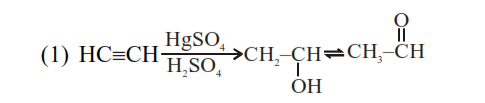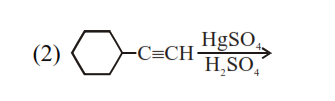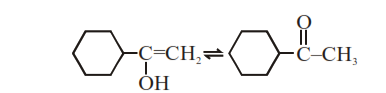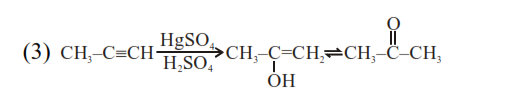Hence $\mathrm{CH}_{3}-\mathrm{CH}_{2}-\mathrm{CHO}$ cannot be form.